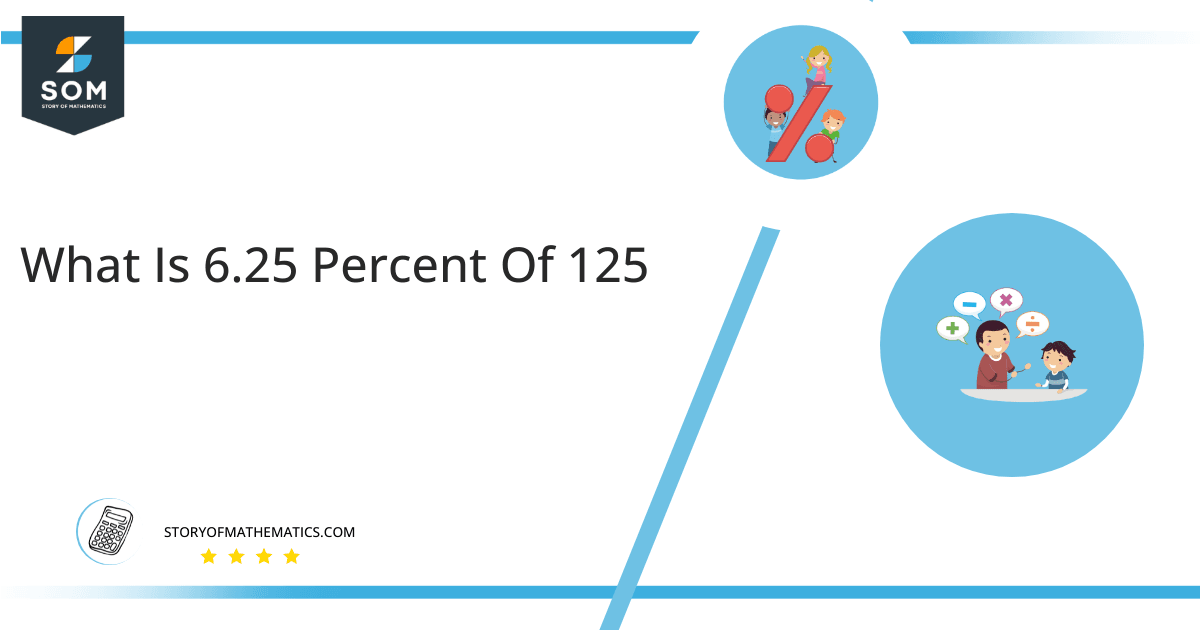# What Is 6.25 Percent of 125+ Solution with Free Steps?The 6.25 percent of 125 is equal to 7.8125. It can be easily calculated by dividing 6.25 by 100 and multiplying the answer with 125 to get 7.8125.

The easiest way to get this answer is by solving a simple mathematical problem of percentage. You need to find 6.25% of 125 for some sale or real-life problem. Divide 6.25 by 100, multiply the answer with 125, and get the 6.25% of 125 value in seconds.

This article will explain the full process of finding any percentage value from any given quantity or number with easy and simple steps.

## What Is 6.25 percent of 125?

The 6.25 percent of 125 is 7.8125.

The percentage can be understood with a simple explanation. Take 125, and divide it into 100 equal parts. The 6.25 number of parts from the total 100 parts is called 6.25 percent, which is 7.8125 in this example.

## How To Calculate 6.25 percent of 125?

You can find 6.25 percent of 125 by some simple mathematical steps explained below.### Step 1

Firstly, depict 6.25 percent of 125 as a fractional multiple as shown below:

6.25% x 125

### Step 2

The percentage sign % means percent, equivalent to the fraction of 1/100.

Substituting this value in the above formula:

= (6.25/100) x 125

### Step 3

Using the algebraic simplification process, we can arithmetically manipulate the above equation as follows:

= (6.25 x 125) / 100

= 781.25 / 100

= 7.8125This percentage can be represented on a pie chart for visualization. Let us suppose that the whole pie chart represents the 125 value. Now, we find 6.25 percent of 125, which is 7.8125. The area occupied by the 7.8125 value will represent the 6.25 percent of the total 125 value. The remaining region of the pie chart will represent 93.75 percent of the total 125 value. The 100% of 125 will cover the whole pie chart as 125 is the total value.

Any given number or quantity can be represented in percentages to better understand the total quantity. The percentage can be considered a quantity that divides any number into hundred equal parts for better representation of large numbers and understanding.

Percentage scaling or normalization is a very simple and convenient method of representing numbers in relative terms. Such notations find wide application in many industrial sectors where the relative proportions are used.